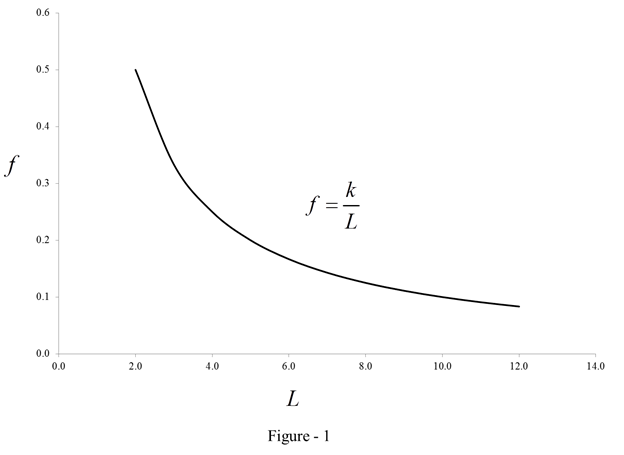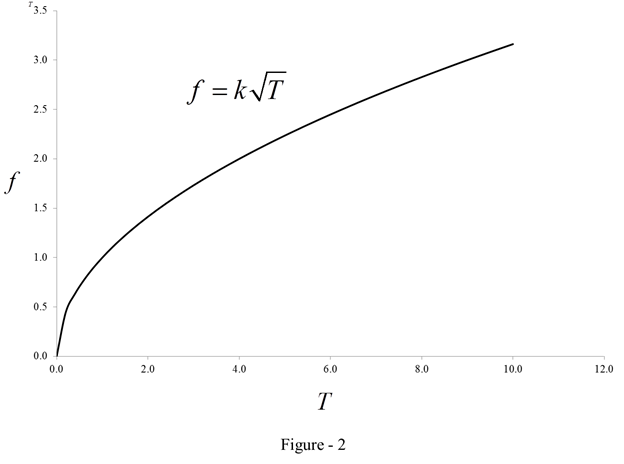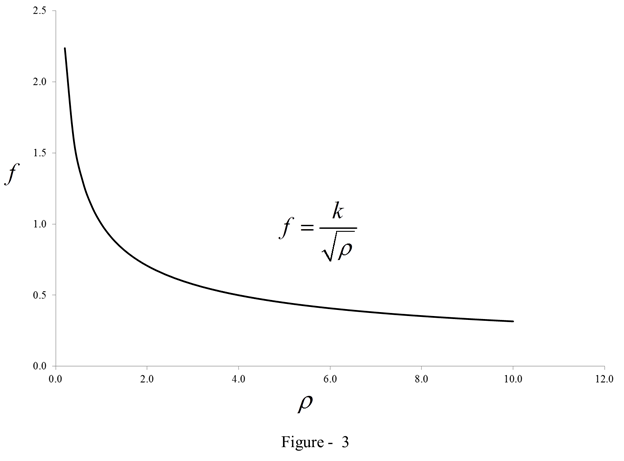# The rate of change of the frequency with respect to the length (When T and ρ are constant)### Single Variable Calculus: Concepts...

4th Edition
James Stewart
Publisher: Cengage Learning
ISBN: 9781337687805### Single Variable Calculus: Concepts...

4th Edition
James Stewart
Publisher: Cengage Learning
ISBN: 9781337687805

#### Solutions

Chapter 3.8, Problem 28E

(a)

(i)

To determine

## To find: The rate of change of the frequency with respect to the length (When T and ρ are constant)

Expert Solution

The rate of change of frequency with respect to length is 12L2TP.

### Explanation of Solution

Given:

The frequency of vibrations of a vibrating violin string is as given below.

f=12LTρ (1)

Calculation:

Calculate the rate of change of frequency with respect to length when T and ρ are constant.

Differentiate the equation (1) with respect to L.

dfdL=ddL(12LTρ)=12Tρ(d(L)1dL)=12Tρ(L2)=12Tρ(1L2)

dfdL=12L2Tρ (2)

Thus, the rate of change of frequency with respect to length is dfdL=12L2Tρ.

(i)

To determine

### To find: The rate of change of the frequency with respect to the length (When T and ρ are constant)

Expert Solution

The rate of change of frequency with respect to length is 12L2TP.

### Explanation of Solution

Given:

The frequency of vibrations of a vibrating violin string is as given below.

f=12LTρ (1)

Calculation:

Calculate the rate of change of frequency with respect to length when T and ρ are constant.

Differentiate the equation (1) with respect to L.

dfdL=ddL(12LTρ)=12Tρ(d(L)1dL)=12Tρ(L2)=12Tρ(1L2)

dfdL=12L2Tρ (2)

Thus, the rate of change of frequency with respect to length is dfdL=12L2Tρ.

(ii)

To determine

### To find: The rate of change of the frequency with respect to the tension (When L and ρ are constant)

Expert Solution

The rate of change of frequency with respect to tension is 14LTρ.

### Explanation of Solution

Calculate the rate of change of frequency with respect to tension when L and ρ are constant.

Differentiate the equation (1) with respect to T.

dfdT=ddT(12LTρ)=12Lρ(d(T)dT)=12Lρ(d(T1/2)dT)

dfdT=12Lρ(T1/22)=12Lρ(12T)

dfdT=14LTρ (3)

Thus, the rate of change of frequency with respect to tension is dfdT=14LTρ.

(iii)

To determine

### To find: The rate of change of the frequency with respect to the linear density (When L and T are constant)

Expert Solution

The rate of change of frequency with respect to linear density is T4Lρ3/2.

### Explanation of Solution

Calculate the rate of change of frequency with respect to linear density when T and L are constant.

Differentiate the equation (1) with respect to ρ.

dfdρ=ddρ(12LTρ)=T2L(d(ρ1/2)dρ)=T2L(12ρ3/2)

dfdρ=T4Lρ3/2 (4)

Thus, the rate of change of frequency with respect to linear density is dfdρ=T4Lρ3/2.

(b)

(i)

To determine

### To find: To determine the behavior of pitch of the note “when the effective length of a string is decreased by placing a finger on the string is decreased by placing a finger on the string so a shorter portion of the string vibrates”.

Expert Solution

The pitch of the note is higher.

### Explanation of Solution

Determine the behavior of the pitch note when the effective length of a string is decreased.

Sketch the curve.

Calculate the value of f using the relation.

f=kL

Here f is the frequency of vibration and L is the length.

Substitute 1 for k and 2 for L in the above equation.

f=12=0.5

Similarly, calculate the remaining values of f.

Calculate and tabulate the remaining values of f as shown in table (1).

 k L f=kL 1 2 0.5 1 3 0.3 1 4 0.25 1 5 0.2 1 6 0.17 1 7 0.14 1 8 0.12 1 9 0.11 1 10 0.1 1 11 0.09

Graph:

Plot the graph using table (1) as shown in figure (1).Refer the figure (1).

Here the frequency of vibration f is increasing when the length L decreases. Thus, the condition dfdL<0 is true. Hence the pitch is in higher note.

(i)

To determine

### To find: To determine the behavior of pitch of the note “when the effective length of a string is decreased by placing a finger on the string is decreased by placing a finger on the string so a shorter portion of the string vibrates”.

Expert Solution

The pitch of the note is higher.

### Explanation of Solution

Determine the behavior of the pitch note when the effective length of a string is decreased.

Sketch the curve.

Calculate the value of f using the relation.

f=kL

Here f is the frequency of vibration and L is the length.

Substitute 1 for k and 2 for L in the above equation.

f=12=0.5

Similarly, calculate the remaining values of f.

Calculate and tabulate the remaining values of f as shown in table (1).

 k L f=kL 1 2 0.5 1 3 0.3 1 4 0.25 1 5 0.2 1 6 0.17 1 7 0.14 1 8 0.12 1 9 0.11 1 10 0.1 1 11 0.09

Graph:

Plot the graph using table (1) as shown in figure (1).Refer the figure (1).

Here the frequency of vibration f is increasing when the length L decreases. Thus, the condition dfdL<0 is true. Hence the pitch is in higher note.

(ii)

To determine

### To find: To determine the behavior of pitch of the note “When the tension is increased by turning a tuning peg.”.

Expert Solution

The pitch of the note is higher.

### Explanation of Solution

Determine the behavior of the pitch note when the tension is increased by turning a tuning peg.

Sketch the curve.

Calculate the value of f using the relation.

f=kT

Here f is the frequency of vibration and T is the tension.

Substitute 1 for k and 0 for T in the above equation.

f=kT=0

Similarly, calculate the remaining values of f.

Calculate and tabulate the remaining values of f as shown in table (2).

 k T f=kT 1 0.0 0.0 1 0.2 0.4 1 0.4 0.6 1 0.6 0.8 1 0.8 0.9 1 1.0 1.0 1 1.2 1.1 1 1.4 1.2 1 1.6 1.3 1 1.8 1.3 1 2.0 1.4 1 2.2 1.5 1 2.4 1.5 1 2.6 1.6 1 2.8 1.7 1 3.0 1.7 1 3.2 1.8 1 3.4 1.8 1 3.6 1.9 1 3.8 1.9 1 4.0 2.0 1 4.2 2.0 1 4.4 2.1 1 4.6 2.1 1 4.8 2.2 1 5.0 2.2 1 5.2 2.3 1 5.4 2.3 1 5.6 2.4 1 5.8 2.4 1 6.0 2.4 1 6.2 2.5 1 6.4 2.5 1 6.6 2.6 1 6.8 2.6 1 7.0 2.6 1 7.2 2.7 1 7.4 2.7 1 7.6 2.8 1 7.8 2.8 1 8.0 2.8 1 8.2 2.9 1 8.4 2.9 1 8.6 2.9 1 8.8 3.0 1 9.0 3.0 1 9.2 3.0 1 9.4 3.1 1 9.6 3.1 1 9.8 3.1 1 10.0 3.2

Graph:

Plot the graph using table (2) shown in figure (2).Refer the figure (2).

Here the frequency of vibration f is increasing when the tension T decreases. Thus, the condition dfdT>0 is true. Hence the pitch is in higher note.

(iii)

To determine

### To find: To determine the behavior of pitch of the note “When the linear density is increased by switching to another string”.

Expert Solution

The pitch of the note is lower.

### Explanation of Solution

Determine the behavior of the pitch note when the linear density is increased by switching to another string.

Sketch the curve.

Calculate the value of f using the relation.

f=kρ

Here f is the frequency of vibration and ρ is linear density.

Substitute 1 for k and 0 for T in the above equation.

f=kρ=11=1

Similarly, calculate the remaining values of f.

Calculate and tabulate the remaining values of f as shown in table (3).

 k ρ f=kρ 1 0.2 2.2 1 0.4 1.6 1 0.6 1.3 1 0.8 1.1 1 1.0 1.0 1 1.2 0.9 1 1.4 0.8 1 1.6 0.8 1 1.8 0.7 1 2.0 0.7 1 2.2 0.7 1 2.4 0.6 1 2.6 0.6 1 2.8 0.6 1 3.0 0.6 1 3.2 0.6 1 3.4 0.5 1 3.6 0.5 1 3.8 0.5 1 4.0 0.5 1 4.2 0.5 1 4.4 0.5 1 4.6 0.5 1 4.8 0.5 1 5.0 0.4 1 5.2 0.4 1 5.4 0.4 1 5.6 0.4 1 5.8 0.4 1 6.0 0.4 1 6.2 0.4 1 6.4 0.4 1 6.6 0.4 1 6.8 0.4 1 7.0 0.4 1 7.2 0.4 1 7.4 0.4 1 7.6 0.4 1 7.8 0.4 1 8.0 0.4 1 8.2 0.3 1 8.4 0.3 1 8.6 0.3 1 8.8 0.3 1 9.0 0.3 1 9.2 0.3 1 9.4 0.3 1 9.6 0.3 1 9.8 0.3 1 10.0 0.3

Graph:

Plot the graph using table (3) as shown in figure (3).